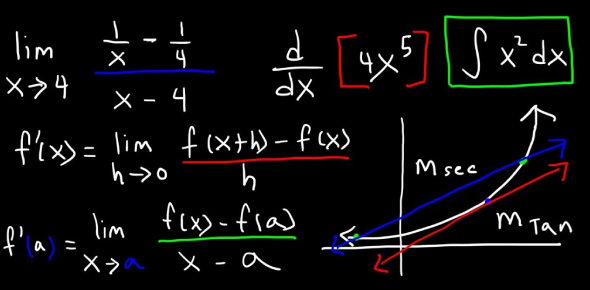# Calculus Quiz Questions With Answers

10 Questions | Attempts: 20501SettingsWe present you here an interesting 'Calculus quiz' that will test your mathematics skills. Calculus is the mathematical study of continuous change, in the same way, that geometry is the study of shape and algebra is the study of generalizations of arithmetic operations. How good are you when it comes to calculus? Take up the quiz below on basic calculus and see if you are in the big league already. So, are you ready to take this test? Let's start then.

• 1.
What is x2 + 1 differentiated with respect to x?
• A.

2x

• B.

X

• C.

X+1

• D.

1

• E.

2x+1

• 2.
What will be the derivative of this function-    'f(x) = 1963'
• A.

0

• B.

1963

• C.

−∞

• D.

None of the above

• 3.
What is the integral of 1 with respect to x?
• A.

0.5x

• B.

0.75x

• C.

X

• D.

1.25x

• E.

2x

• 4.
State whether the given statement is true or false. The second fundamental theorem of calculus states that if F(x) = ∫ ax f(t) dt then F '(x) = f(x).
• A.

True

• B.

False

• 5.
What is x2 + 1 differentiated with respect to y?
• A.

2x

• B.

2x+1

• C.

Xy +1

• D.

0

• E.

(x+1)y

• 6.
What function is integrated to get this graph?
• A.

-x+1

• B.

X-1

• C.

7x

• D.

(x+1)

• E.

2x

• 7.
Here's a theory-based question. Can you name the two men who discovered calculus?
• A.

Newton

• B.

Gottfried Wilhelm Leibniz

• C.

Thomas Edison

• D.

Georg Cantor

• 8.
From the video, what is slope defined as?
• A.

Change in y divided by change in x

• B.

Change in x multiplied by change in x

• C.

Change in y multiplied by change in x

• D.

Change in x divided by change in x

• E.

Change in x divided by change in y

• 9.
What is the limit of 1/(x-1) as x goes to infinity?
• A.

Infinity

• B.

1

• C.

0

• D.

-1

• E.

Negative infinity

• 10.
The limit as x goes to zero of x-1 exists.
• A.

True

• B.

False

## Related TopicsBack to top
×

Wait!
Here's an interesting quiz for you.